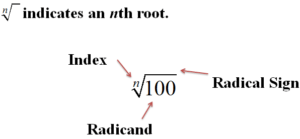Roots, radicands, and radicals are yet another way to express numbers in algebra. In this post, we will go over some basic terms to know.Roots

A square root is a number that is multiplied by itself to get a new number. Below is an exampleIn the example above 5 is the square root of 25. This means that if you multiply 5 by its self you would get 25.

Another term to know is the square. The square is the result of multiplying a number by its self. In the expression above 25 is the square of 5 because you get 25 by multiplying 5 by its self.

Square Roots

Square roots, in particular, have a lot of other ways to be expressed. To understand square roots you need to know what roots, radicands, and radical sign are. Below is a picture of these three parts.The radical sign is simply a sign like multiplication and division are. The radicand is the number you want to simplify by finding a number that when multiplied by itself would equal the value in the radicand. We also call this new number the square root. For example,What the example above means is that the number you can multiply by itself to get 100 is 10.

The index is trickier to understand. It tells you how many times to multiply the number by its self to get the radicand. If no number is there you assume the index is 2. Below is an expression with an index that is not 2.What this expression is saying is that you can multiply 2 by its self 3 times to get eight as you can see below.